Every day I’m shufflin'.
Shufflin', shufflin'.
-- "Party Rock Anthem," LMFAO

The most popular way to mix a deck of cards is the riffle shuffle, which separates the deck into two pieces and interleaves the cards from each piece. Besides being popular with card players, the riffle shuffle is well-known among statisticians because Bayer and Diaconis (1992) showed that it takes seven riffle shuffles to randomize a 52-card deck. This result annoyed many recreational card players because shuffling seven times "slows down the game".

The second most popular shuffling technique is the overhand shuffle. The overhand shuffle is much less efficient at mixing the cards than a riffle shuffle. A result by Pemantle (1989) indicates that at least 50 overhand shuffles are required to adequately mix the deck, but computer experiments (Pemantle, 1989, p. 49) indicate that in many situations 1,000 or more overhand shuffles are required to randomize a deck. Talk about slowing down the game! This article shows how to simulate an overhand shuffle in SAS and visually compares the mixing properties of the riffle and overhand shuffles.

### The overhand shuffle

An overhand shuffle transfers a few cards at a time from the dealer's right hand to his left. The dealer slides a few cards from the top of the deck in his right hand into his left hand. The process is repeated until all cards in his right hand are transferred. Consequently, cards that started near the top of the deck end up near the bottom, and vice versa.

Mathematically, you can model an overhand shuffle by randomly choosing k cut points that separate the deck into k+1 "packets" of contiguous cards. The size of a packet can vary, as can the number of packets. The overhand shuffle reverses the order of the packets while preserving the order of the cards within each packet. When the packet sizes are small, the cards are mixed better than when the packets are bigger.

For example, a deck that contains 10 cards (enumerated 1-10) might be split into five packets such as (1)(234)(5)(678)(9 10), where the parentheses show the cards in each of the packets. In this example, the cut points are the locations after cards 1, 4, 5, and 8. The packets contain 1, 3, 1, 3, and 2 cards. The overhand shuffle reverses the order of the packets, so the deck is reordered as (9 10)(678)(5)(234)(1). This is shown in the following graphic.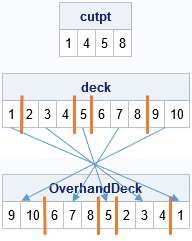In a statistical model of the overhand shuffle, the cut points are assumed to be randomly chosen with probability p from among the N-1 possible locations between adjacent cards. An ideal overhand shuffle uses p = 1/2, which results in packets that are typically sized, 1, 2, and 3 (the packet size is geometrically distributed). However, among amateurs, the probability is much lower and an overhand shuffle reorders only a few large packets, which can lead to poor mixing of the cards.

### Simulate an overhand shuffle

I previously showed how to simulate a riffle shuffle in the SAS/IML language. The following program shows how to simulate an overhand shuffle. The deck of cards (represented as a vector of integers with the values 1 to N) is passed in, as is the probability of choosing a cut point. To test the program, I pass in a deck that has 10 cards enumerated by the integers 1–10. The new order of the deck was shown in the previous section.

```proc iml; call randseed(1234);   /* Simulate overhand shuffle. Randomly split deck into k packets. Reverse the order of the packets. Each position between cards is chosen to be a cut point with probability p. (Default: p=0.5) */ start Overhand(_deck, p=0.5); deck = colvec(_deck); /* make sure input is column vector */ N = nrow(deck); /* number of cards in deck */ b = randfun(N-1, "Bernoulli", p); /* randomly choose cut points */ if all(b=0) then return _deck; /* no cut points were chosen */ cutpt = loc(b=1); /* cut points in range 1:n-1 */ cutpt = 0 || cutpt || N; /* append 0 and n */ rev = n - cutpt + 1; /* reversed positions */   newDeck = j(N, 1, .); do i = 1 to ncol(cutpt)-1; /* for each packet... */ R = (1 + cutpt[i]):cutpt[i+1]; /* positions of cards in right hand */ L = rev[i+1]:(rev[i]-1); /* new positions in left hand */ newDeck[L] = deck[R]; /* transfer packet from right to left hand */ end; return shape(newDeck, nrow(_deck), ncol(_deck)); /* return same shape as input */ finish;   deck = 1:10; OverhandDeck = Overhand(deck, 0.5); print deck; print OverhandDeck;```

### Simulate many overhand shuffles

Let's now consider the standard 52-card deck, represented by the integers 1–52. The following loop performs 10 overhand shuffles (p = 0.5) and uses a heat map to show the order of the cards after each shuffle:

```N = 52; /* standard 52-card deck */ deck = 1:N; /* initial order */ numShuffles = 10; /* number of times to shuffle cards */   OverDeck = j(numShuffles+1, N); OverDeck[1,] = deck; do i = 2 to numShuffles + 1; OverDeck[i,] = Overhand(OverDeck[i-1,]); end;   ramp = palette("YLORBR",9); call heatmapcont(OverDeck) colorramp=ramp yvalues=0:numShuffles title="Positions of 52 Cards After Overhand Shuffles";```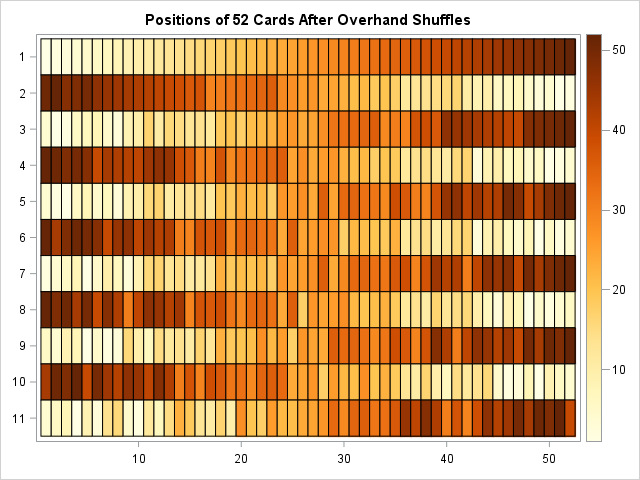The cell colors in the heat map correspond to the initial position of the cards: light colors indicate cards that are initially near the top of the deck and dark colors represent cards that are initially near the bottom of the deck. The first row shows the initial positions of the cards in the deck. The second row shows the cards after one overhand shuffle. The third row shows the cards after two overhand shuffles, and so on. It is interesting to see how the pattern changes if you use p=0.25 or p=0.1. Try it!

One feature that is apparent in the image is that the relative positions of cards depend on whether you have performed an even or an odd number of overhand shuffles. After an odd number of shuffles, the top of the deck contains many cards that were initially at the bottom, and the bottom of the deck contains cards that were initially at the top. After an even number of shuffles, the top of the deck contains many cards that were initially at the top. Another apparent feature is that this process does not mix the cards very well. The last row in the heat map indicates that after 10 overhand shuffles, many cards are not very far from their initial positions. Furthermore, many cards that were initially next to each other are still within a few positions of each other.

Maybe additional overhand shuffles will address these deficiencies? The following program simulates 100 overhand shuffles. To aid in the visualization, the heat map shows only the results after 2, 4, 6, ..., and 100 shuffles (the even numbers):

```deck = 1:N; /* reset initial order */ numShuffles = 100; /* number of times to shuffle cards */ numEvenShuffles = numShuffles / 2; OverDeck = j(numEvenShuffles+1, N);   OverDeck[1,] = deck; /* save only the EVEN shuffles */ do i = 2 to numEvenShuffles + 1; OverDeck[i,] = Overhand(Overhand( OverDeck[i-1,] )); /* 2 calls */ end;   call heatmapcont(OverDeck) colorramp=ramp yvalues=0:numEvenShuffles title="Positions of 52 Cards After EVEN Overhand Shuffles";```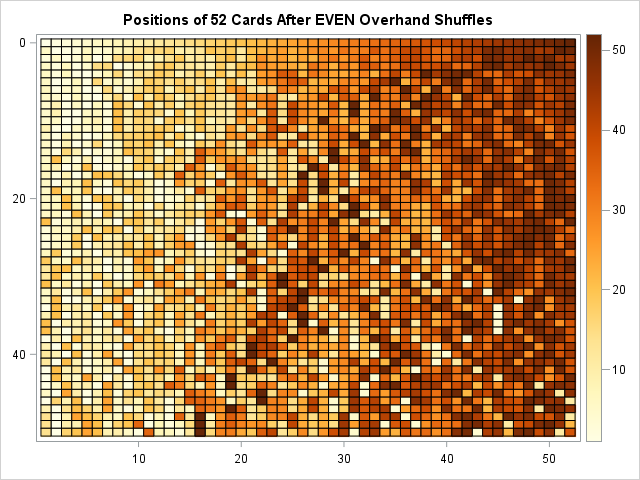The heat map indicates that the deck is not well-mixed even after 100 overhand shuffles. Look at the last row in the heat map, which shows the positions of the cards in the deck colored by their initial position. There are a small number of light-colored cells on the right side of the heat map (cards that were initially on top; now near the bottom), and a small number of dark-colored cells on the left side of the heat map (cards that were initially on the bottom; now near the top). However, in general, the left side of the heat map contains mostly light-colored cells and the right side contains mostly dark-colored cells, which indicates that cards that have not moved far from their initial positions.

If you use smaller values of p (that is, the average packet size is larger), you will observe different patterns. The theory says that large packets cause nearby cards to tend to stay together for many shuffles. Jonasson (2006) was able to prove that if a deck has N cards, then O(N2 log(N)) overhand shuffles are required to randomize the deck. This compares with (3/2) log2(N) for the riffle shuffle, which is presented in the next section.

### A comparison with the riffle shuffle

The riffle shuffle, which randomizes a deck after about seven shuffles. You can simulate a riffle shuffle in SAS and create a similar heat map. The following heat map shows the order of a 52-card deck after each of 10 riffle shuffles: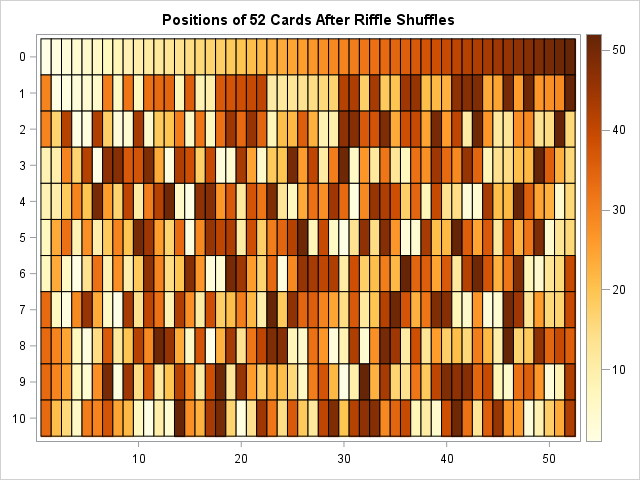The heat map shows how quickly a riffle shuffle randomizes a deck. The last row (after 10 riffles) shows that light-colored cells are distributed throughout the deck, as are dark-colored cells and medium-colored cells. Furthermore, this behavior seems to occur after six or seven rows (at least to my untrained eye). I cannot see much difference between the "randomness" in row 6 and row 10. However, theoretically, a card in an earlier row (say, row 5) is more likely to be near cards that were initially close to it. For example, a dark-colored cell in row 5 is more likely to be near another dark-colored cell than would be expected in a randomized deck. However, by the time you perform 7 or more riffle shuffles, two cards that started near each other might be located far apart or might be close to each other. You cannot predict which will occur because the cards have been randomized.

In summary, the overhand shuffle does not mix cards very well. The simulation indicates that an overhand shuffle is not effective at separating cards that begin near each other. Even after 100 overhand shuffles (with p=0.5), the positions of many cards are only slightly different from their initial positions. In contrast, a riffle shuffle is an effective way to randomize a deck in approximately seven shuffles.

The post Shuffling smackdown: Overhand shuffle versus riffle shuffle appeared first on The DO Loop.Have you ever tried to type a movie title by using a TV remote control? Both Netflix and Amazon Video provide an interface (a virtual keyboard) that enables you to use the four arrow keys of a standard remote control to type letters. The letters are arranged in a regular grid and to navigate from one letter to another can require you to press the arrow keys many times. Fortunately, the software displays partial matches as you choose each letter, so you rarely need to type the entire title. Nevertheless, I was curious: Which interface requires the fewest number of key presses (on average) to type a movie title by using only the arrow keys?

### The layout of the navigation screens

The following images show the layout of the navigation screen for Netflix and for Amazon Video.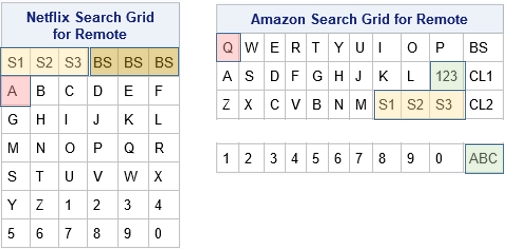The Netflix grid has 26 letters and 10 numbers arranged in a 7 x 6 grid. The letters are arranged in alphabetical order. The first row contains large keys for the Space character (shown in a light yellow color) and the Backspace key (dark yellow). Each of those keys occupies three columns of the grid, which means that you can get to the Space key by pressing Up Arrow from the A, B, or C key. When you first arrive at the Netflix navigation screen, the cursor is positioned on the A key (shown in pink).

The letters in the Amazon Video grid are arranged in a 3 x 11 grid according to the standard QWERTY keyboard layout. When you first arrive at the navigation screen, the cursor is positioned on the Q key. The Space character can be typed by using a large key in the last row (shown in a light yellow color) that spans columns 8, 9, and 10. The Space character can be accessed from the M key (press Right Arrow) or from the K, L, or '123' keys on the second row. The '123' key (shown in green) navigates to a second keyboard that contains numbers and punctuation. The numbers are arranged in a 1 x 11 grid. When you arrive at that keyboard, the cursor is on the 'ABC' key, which takes you back to the keyboard that contains letters. (Note: The real navigation screen places the '123' key under the 0 key. However, the configuration in the image is equivalent because in each case you must press one arrow key to get to the 0 (zero) key.) For simplicity, this article ignores punctuation in movie titles.

### Which navigation interface is more efficient?

I recently wrote a mathematical discussion about navigating through grids by moving only Up, Down, Left, and Right. The article shows that nearly square grids are more efficient than short and wide grids, assuming that the letters that you type are chosen at random. A 7 x 6 grid requires an average of 4.23 key presses per character whereas a 4 x 11 grid requires an average of 4.89 key presses per character. Although the difference might not seem very big, the average length of a movie title is about 15 characters (including spaces). For a 15-character title, the mathematics suggests that using the Netflix interface requires about 10 fewer key presses (on average) than the Amazon Video interface.

If you wonder why I did not include the Hulu interface in this comparison, it is because the Hulu "keyboard" is a 1 x 28 grid that contains all letters and the space and backspace keys. Theory predicts an average of 9.32 key presses per character, which is almost twice as many key presses as for the Netflix interface.

### Comparing two interfaces for selecting movie titles

You might wonder how well this theoretical model matches reality. Movie titles are not a jumble of random letters! How do the Netflix and Amazon Video interfaces compare when they are used to type actual movie titles?

To test this question, I downloaded the titles of 1,000 highly-rated movies. I wrote a SAS program that calculates the number of the arrow keys that are needed to type each movie title for each interface. This section summarizes the results.

The expression "press the arrow key," is a bit long, so I will abbreviate it as "keypress" (one word). The "number of times that you need to press the arrow keys to specify a movie title" is similarly condensed to "the number of keypresses."

For these 1,000 movie titles, the Netflix interface requires an average of 50.9 keypresses per title or 3.32 keypresses per character. the Amazon Video interface requires an average of 61.4 keypresses per title or 4.01 keypresses per character. Thus, on average, the Netflix interface requires 10.56 fewer keypresses per title, which closely agrees with the mathematical prediction that consider only the shape of the keyboard interface. A paired t test indicates that the difference between the means is statistically significant. The difference between medians is similar: 45 for the Netflix interface and 56 for the Amazon interface.

The following comparative histogram (click to enlarge) shows the distribution of the number of keypresses for each of the 1,000 movie titles for each interface. The upper histogram shows that most titles require between 30 and 80 keypresses in the Amazon interface, with a few requiring more than 140 keypresses. In contrast, the lower histogram indicates that most titles require between 20 and 60 keypresses in the Netflix interface; relatively fewer titles require more than 140 keypresses.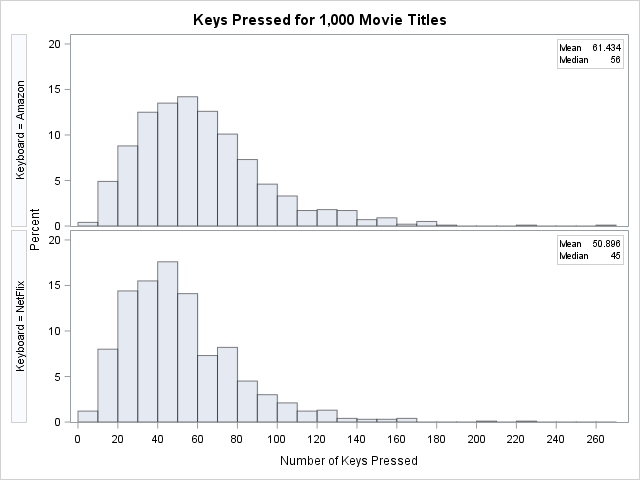You can also use a scatter plot to compare the number of keypresses that are required for each interface. Each marker in the following scatter plot shows the number of keypresses for a title in the Amazon interface (horizontal axis) and the Netflix interface (vertical axis). Markers that are below and to the right of the diagonal (45-degree) line are titles for which the Netflix interface requires fewer keypresses. Markers that are above and to the left of the diagonal line are titles for which the Amazon interface is more efficient. You can see that most markers are below the diagonal line. In fact, 804 titles require fewer keypresses in the Netflix interface, only 177 favor the Amazon interface, and 19 require the same number of keypresses in both interfaces. Clearly, the Netflix layout of the virtual keyboard is more efficient for specifying movie titles.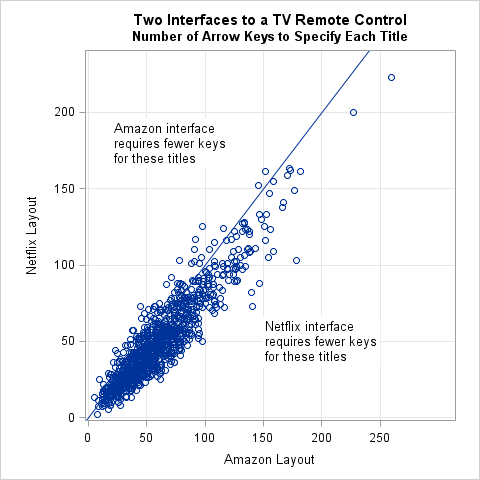### Movie titles that require the most and the fewest key presses

The scatter plot and histograms reveal that there are a few movies whose titles require many keypresses. Here is a list of the 10 titles that require the most keypresses when using the Amazon interface: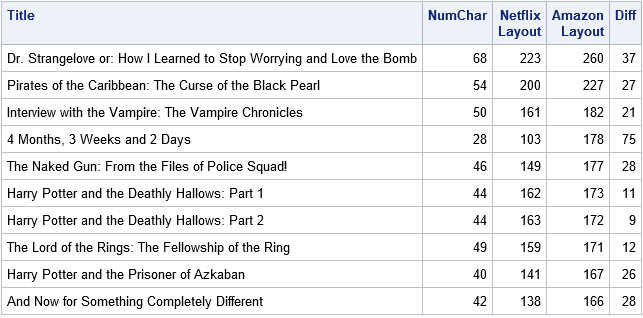Most of the titles are long. However, one (4 Months, 3 Weeks and 2 Days) is not overly long but instead requires shifting back and forth between the two keyboards in the Amazon interface. That results in a large number of keypresses in the Amazon interface (178) and a large difference between the keypresses required by each interface. In fact, the absolute difference for that title (75) is the largest difference among the 1,000 titles.

You can also look at the movie titles that require few keypresses. The following table shows titles that require fewer than 10 keypresses in either interface. The titles that require the fewest keypresses in the Netflix interface are M, Moon, PK, and Up. The titles that require the fewest keypresses in the Amazon interface are Saw, M, Creed, and Up. It is interesting that Saw, which has three letters, requires fewer keypresses than M, which has one letter. That is because the S, A, and W letters are all located in the upper left of the QWERTY keyboard whereas the letter M is in the lower left corner of the keyboard. (Recall that the cursor begins on the Q letter in the upper left corner.).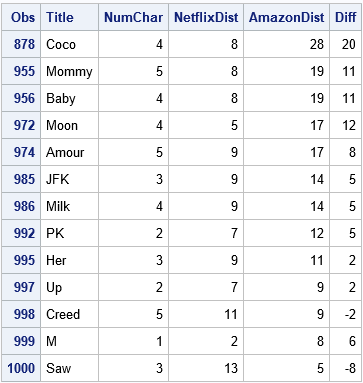### Summary

In summary, both Netflix and Amazon Video provide an interface that enables customers to select movie titles by using the four arrow keys on a TV remote control. The Netflix interface is a 7 x 6 grid of letters; the Amazon interface is a 3 x 11 QWERTY keyboard and a separate keyboard for numbers. In practice, both interfaces display partial matches and you only need to type a few characters. However, it is interesting to statistically compare the interfaces in terms of efficiency. For a set of 1,000 movie titles, the Netflix interface requires, on average, 10.6 fewer keypresses than the Amazon interface to completely type the titles. This article also lists the movie titles that require the most and the fewest number of key presses.

If you would like to duplicate or extend this analysis, you can download the SAS program that contains the data.

The post Two interfaces for typing text by using a TV remote control appeared first on The DO Loop.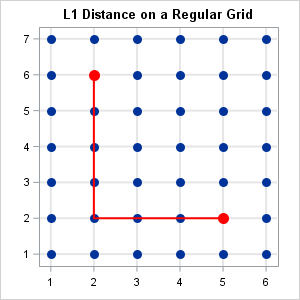Given a rectangular grid with unit spacing, what is the expected distance between two random vertices, where distance is measured in the L1 metric? (Here "random" means "uniformly at random.") I recently needed this answer for some small grids, such as the one to the right, which is a 7 x 6 grid. The graph shows that the L1 distance between the points (2,6) and (5,2) is 7, the length of the shortest path that connects the two points. The L1 metric is sometimes called the "city block" or "taxicab" metric because it measures the distance along the grid instead of "as the bird flies," which is the Euclidean distance.

The answer to the analogous question for the continuous case (a solid rectangle) is difficult to compute. The main result is that the expected distance is less than half of the diameter of the rectangle. In particular, among all rectangles of a given area, a square is the rectangle that minimizes the expected distance between random points.

Although I don't know a formula for the expected distance on a discrete regular grid, the grids in my application were fairly small so this article shows how to compute all pairwise distances and explicitly find the average (expected) value. The DISTANCE function in SAS/IML makes the computation simple because it supports the L1 metric. It is also simple to perform computer experiments to show that among all grids that have N*M vertices, the grid that is closest to being square minimizes the expected L1 distance.

### L1 distance on a regular grid

An N x M grid contains NM vertices. Therefore the matrix of pairwise distances is NM x NM. Without loss of generality, assume that the vertices have X coordinates between 1 and N and Y coordinates between 1 and M. Then the following SAS/IML function defines the distance matrix for vertices on an N x M grid. To demonstrate the computation, the distance matrix for a 4 x 4 grid is shown, along with the average distance between the 16 vertices:

```proc iml; start DistMat(rows, cols); s = expandgrid(1:rows, 1:cols); /* create (x, y) ordered pairs */ return distance(s, "CityBlock"); /* pairwise L1 distance matrix */ finish;   /* test function on a 4 x 4 grid */ D = DistMat(4, 4); AvgDist = mean(colvec(D)); print D[L="L1 Distance Matrix for 4x4 Grid"]; print AvgDist[L="Avg Distance on 4x4 Grid"];```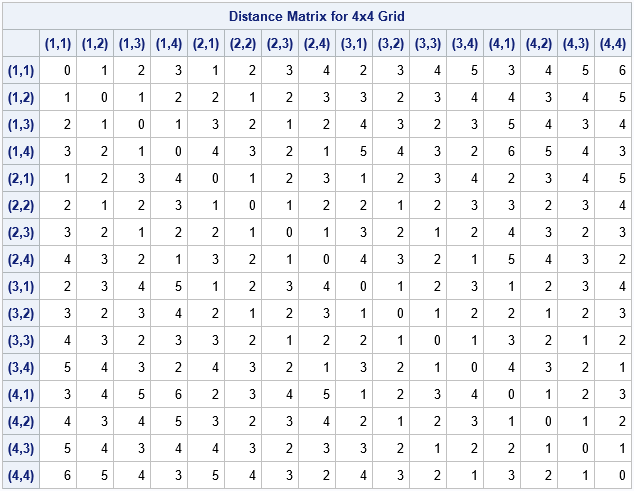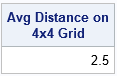For an N x M grid, the L1 diameter of the grid is the L1 distance between opposite corners. That distance is always (N-1)+(M-1), which equates to 6 units for a 4 x 4 grid. As for the continuous case, the expected L1 distance is less than half the diameter. In this case, E(L1 distance) = 2.5.

### A comparison of distances for grids of different aspect ratios

As indicated previously, the expected distance between two random vertices on a grid depends on the aspect ratio of the grid. A grid that is nearly square has a smaller expected distance than a short-and-wide grid that contains the same number of vertices. You can illustrate this fact by computing the distance matrices for grids that each contain 36 vertices. The following computation computes the distances for five grids: a 1 x 36 grid, a 2 x 18 grid, a 3 x 12 grid, a 4 x 9 grid, and a 6 x 6 grid.

```/* average L1 distance on 36 x 36 grid in several configurations */ N=36; rows = {1, 2, 3, 4, 6}; cols = N / rows; AvgDist = j(nrow(rows), 1, .); do i = 1 to nrow(rows); D = DistMat(rows[i], cols[i]); AvgDist[i] = mean(colvec(D)); end; /* show average distance as a decimal and as a fraction */ numer = AvgDist*108; AvgDistFract = char(numer) + " / 108"; print rows cols AvgDist AvgDistFract;```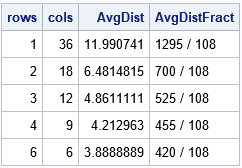The table shows that short-and-wide tables have an average distance that is much greater than a nearly square grid that contains the same number of vertices. When the points are arranged in a 6 x 6 grid, the distance matrix naturally decomposes into a block matrix of 6 x 6 symmetric blocks, where each block corresponds to a row. When the points are arranged in a 3 x 12 grid, the distance matrix decomposes into a block matrix of 12 x 12 blocks The following heat maps visualize the patterns in the distance matrices:

```D = DistMat(6, 6); call heatmapcont(D) title="L1 Distance Between Vertices in a 6 x 6 Grid"; D = DistMat(3, 12); call heatmapcont(D) title="L1 Distance Between Vertices in a 3 x 12 Grid";```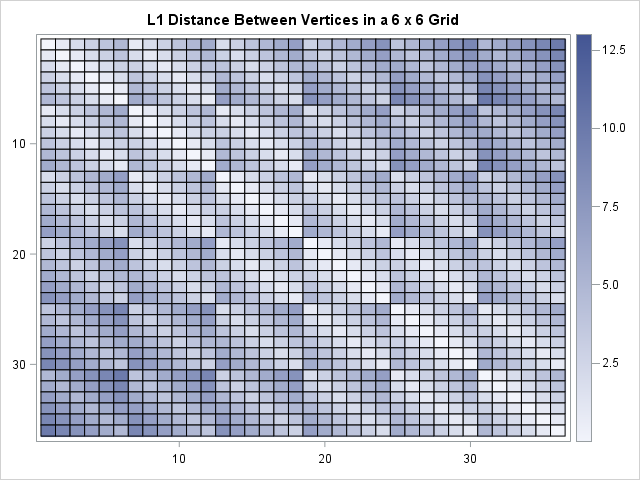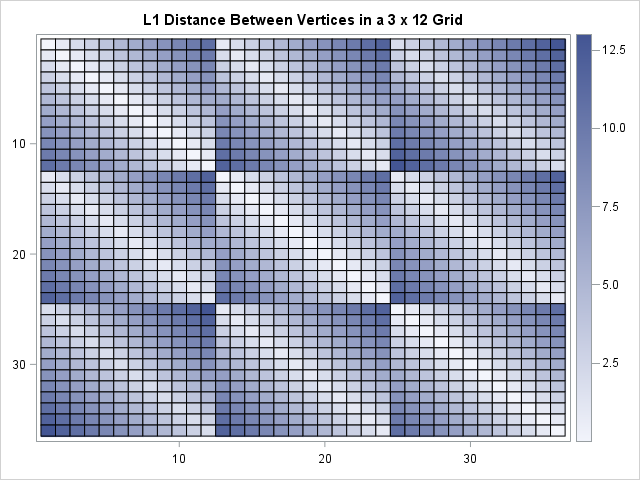### Expected (average) distance versus number of rows

You can demonstrate how the average distance depends on the number of rows by choosing the number of vertices to be a highly composite number such as 5,040. A highly composite number has many factors. The following computation computes the average distance between points on 28 grids that each contain 5,040 vertices. A line chart then displays the average distance as a function of the number of rows in the grid:

```N = 5040; /* 5040 is a highly composite number */ rows = {1, 2, 3, 4, 5, 6, 7, 8, 9, 10, 12, 14, 15, 16, 18, 20, 21, 24, 28, 30, 35, 36, 40, 42, 45, 48, 56, 60, 63, 70}; cols = N / rows; AvgDist = j(nrow(rows), 1, .); do i = 1 to nrow(rows); D = DistMat(rows[i], cols[i]); AvgDist[i] = mean(colvec(D)); end;   title "Average L1 Distance Between 5040 Objects in a Regular Grid"; call series(rows, AvgDist) grid={x y} other="yaxis offsetmin=0 grid;";```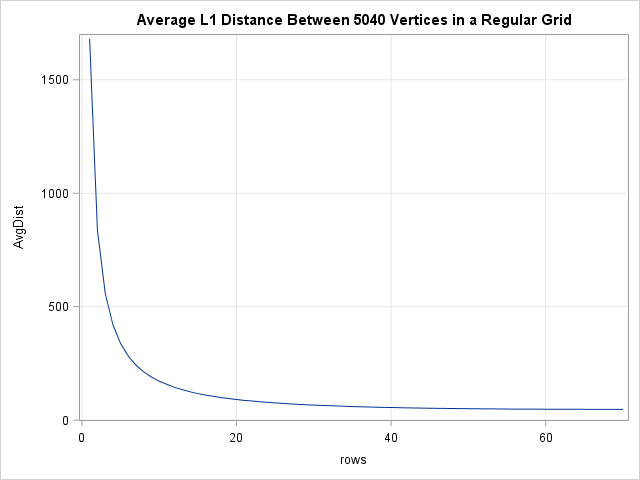Each point on the graph represents the average distance for an N x M grid where NM = 5,040. The horizontal axis displays the value of N (rows). The graph shows that nearly square grids (rows greater than 60) have a much lower average distance than very short and wide grids (rows less than 10). The scale of the graph makes it seem like there is very little difference between the average distance in a grid with 40 versus 70 rows, but that is somewhat of an illusion. A 40 x 126 grid (aspect ratio = 3.15) has an average distance of 55.3; a 70 x 72 grid (aspect ratio = 1.03) has an average distance of 47.3.

### Applications and conclusions

In summary, you can use the DISTANCE function in SAS/IML to explicitly compute the expected L1 distance (the "city block" distance) between random points on a regular grid. You can minimize the average pairwise distance by making the grid as square as possible.

City planning provides a real-world application of the L1 distance. If you are tasked with designing a city with N buildings along a grid, then the average distance between buildings is smallest when the grid is square. Of course, in practice, some buildings have a higher probability of being visited than others (such as a school or a grocery). You should position those buildings in the center of town to shorten the average distance that people travel.

The post Distances on rectangular grids appeared first on The DO Loop.In the SAS/IML language, you can only concatenate vectors that have conforming dimensions. For example, to horizontally concatenate two vectors X and Y, the symbols X and Y must have the same number of rows. If not, the statement Z = X || Y will produce an error: `ERROR: Matrices do not conform to the operation.`

The other day I wanted to concatenate multiple vectors of different sizes into a single matrix. I decided to use missing values to pad the "short" vectors. To make the operation reusable, I developed an IML module that accepts up to 16 different optional arguments. The module uses a few advanced techniques. This article presents the module and discusses programming techniques that you can use to systematically process all arguments passed to a SAS/IML module. Even if you never need to use the module, the techniques that implement the module are useful.

I wrote the module because I wanted to store multiple statistics of different sizes in a single matrix. For example, you can use this technique to store the data, parameter estimates, and the result of a hypothesis test. You can use a list to store the values, but for my application a matrix was more convenient. I've used a similar construction to store an array of matrices in a larger matrix.

### A module to concatenate vectors of different lengths

Although "parameter" and "argument" are often used interchangeably, for this article I will try to be consistent by using the following definitions. A parameter is a local variable in the declaration of the function. An argument is the actual value that is passed to function. Parameters are known at compile time and never change; arguments are known at run time and are potentially different every time the function is called.

Let's first see what the module does, then we'll discuss how it works. The module is defined as follows:

```proc iml; /* Pack k vectors into a matrix, 1 <= k <= 16. Each vector becomes a column. The vectors must be all numeric or all character. */ start MergeVectors(X1, X2=, X3=, X4=, X5=, X6=, X7=, X8=, X9=, X10=, X11=, X12=, X13=, X14=, X15=, X16= ); ParmList = "X1":"X16"; /* Names of params. Process in order. */ done = 0; /* flag. Set to 1 when empty parameter found */ type = type(X1); /* type of first arg; all args must have this type */ maxLen = 0; /* for character args, find longest LENGTH */ N = 0; /* find max number of elements in the args */   /* 1. Count args and check for consistent type (char/num). Stop at first empty arg */ do k = 1 to ncol(ParmList) until(done); arg = value( ParmList[k] ); /* get value from name */ done = IsEmpty(arg); /* if empty matrix, then exit loop */ if ^done then do; /* if not empty matrix... */ if type(arg)^= type then /* check type for consistency */ STOP "ERROR: Arguments must have the same type"; maxLen = max(maxLen,nleng(arg)); /* save max length of char matrices */ N = max(N,prod(dimension(arg))); /* save max number of elements */ end; end;   numArgs = k - 1; /* How many args were supplied? */ if type="N" then init = .; /* if args are numeric, use numeric missing */ else init = BlankStr(maxLen); /* if args are character, use character missing */ M = j(N, numArgs, init); /* allocate N x p matrix of missing values */   /* 2. Go through the args again. Fill i_th col with values of i_th arg */ do i = 1 to numArgs; arg = value( ParmList[i] ); /* get i_th arg */ d = prod(dimension(arg)); /* count number of elements */ M[1:d,i] = arg[1:d]; /* copy into the i_th column */ end; return( M ); /* return matrix with args packed into cols */ finish;   /* test the module */ M = MergeVectors(-1:1, T(1:4), 0:1); print M;```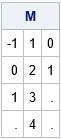In the example, the function is passed three arguments of different sizes. The function returns a matrix that has three columns. The i_th column contains the values of the i_th parameter. The matrix contains four rows, which is the number of elements in the second argument. Missing values are used to pad the columns that have fewer than four elements.

### How to process all arguments to a function

The module in the previous section is defined to take one required parameter and 15 optional parameters. The arguments to the function are processed by using the following techniques:

• The ParmList vector contains the names of the parameters: ParmList = "X1":"X16".
• The module loops over the parameters and uses the VALUE function to get the value of the argument that is passed in.
• The ISEMPTY function counts the number of arguments that are passed in. At the same time, the module finds the maximum number of elements in the arguments.
• The module allocates a result matrix, M, that initially contains all missing values. The module iterates over the arguments and fills the columns of M with their values.

In summary, it is sometimes useful to pack several vectors of different sizes into a single matrix. But even if you never need this functionality, the structure of this module shows how to process an arbitrary number of optional arguments where each argument is processed in an identical manner.

The post Store vectors of different lengths in a matrix appeared first on The DO Loop.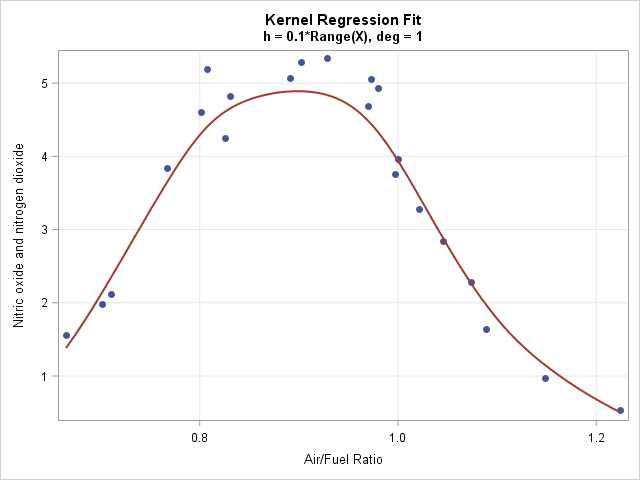A SAS programmer recently asked me how to compute a kernel regression in SAS. He had read my blog posts "What is loess regression" and "Loess regression in SAS/IML" and was trying to implement a kernel regression in SAS/IML as part of a larger analysis. This article explains how to create a basic kernel regression analysis in SAS. You can download the complete SAS program that contains all the kernel regression computations in this article.

A kernel regression smoother is useful when smoothing data that do not appear to have a simple parametric relationship. The following data set contains an explanatory variable, E, which is the ratio of air to fuel in an engine. The dependent variable is a measurement of certain exhaust gasses (nitrogen oxides), which are contributors to the problem of air pollution. The scatter plot to the right displays the nonlinear relationship between these variables. The curve is a kernel regression smoother, which is developed later in this article.

```data gas; label NOx = "Nitric oxide and nitrogen dioxide"; label E = "Air/Fuel Ratio"; input NOx E @@; datalines; 4.818 0.831 2.849 1.045 3.275 1.021 4.691 0.97 4.255 0.825 5.064 0.891 2.118 0.71 4.602 0.801 2.286 1.074 0.97 1.148 3.965 1 5.344 0.928 3.834 0.767 1.99 0.701 5.199 0.807 5.283 0.902 3.752 0.997 0.537 1.224 1.64 1.089 5.055 0.973 4.937 0.98 1.561 0.665 ;```

### What is kernel regression?

Kernel regression was a popular method in the 1970s for smoothing a scatter plot. The predicted value, ŷ0, at a point x0 is determined by a weighted polynomial least squares regression of data near x0. The weights are given by a kernel function (such as the normal density) that assigns more weight to points near x0 and less weight to points far from x0. The bandwidth of the kernel function specifies how to measure "nearness." Because kernel regression has some intrinsic problems that are addressed by the loess algorithm, many statisticians switched from kernel regression to loess regression in the 1980s as a way to smooth scatter plots.

Because of the intrinsic shortcomings of kernel regression, SAS does not have a built-in procedure to fit a kernel regression, but it is straightforward to implement a basic kernel regression by using matrix computations in the SAS/IML language. The main steps for evaluating a kernel regression at x0 are as follows:

1. Choose a kernel shape and bandwidth (smoothing) parameter: The shape of the kernel density function is not very important. I will choose a normal density function as the kernel. A small bandwidth overfits the data, which means that the curve might have lots of wiggles. A large bandwidth tends to underfit the data. You can specify a bandwidth (h) in the scale of the explanatory variable (X), or you can specify a value, H, that represents the proportion of the range of X and then use h = H*range(X) in the computation. A very small bandwidth can cause the kernel regression to fail. A very large bandwidth causes the kernel regression to approach an OLS regression.
2. Assign weights to the nearby data: Although the normal density is infinite in support, in practice, observations farther than five bandwidths from x0 get essentially zero weight. You can use the PDF function to easily compute the weights. In the SAS/IML language, you can pass a vector of data values to the PDF function and thus compute all weights in a single call.
3. Compute a weighted regression: The predicted value, ŷ0, of the kernel regression at x0 is the result of a weighted linear regression where the weights are assigned as above. The degree of the polynomial in the linear regression affects the result. The next section shows how to compute a first-degree linear regression; a subsequent section shows a zero-degree polynomial, which computes a weighted average.

### Implement kernel regression in SAS

To implement kernel regression, you can reuse the SAS/IML modules for weighted polynomial regression from a previous blog post. You use the PDF function to compute the local weights. The KernelRegression module computes the kernel regression at a vector of points, as follows:

```proc iml; /* First, define the PolyRegEst and PolyRegScore modules https://blogs.sas.com/content/iml/2016/10/05/weighted-regression.html See the DOWNLOAD file. */   /* Interpret H as "proportion of range" so that the bandwidth is h=H*range(X) The weight of an observation at x when fitting the regression at x0 is given by the f(x; x0, h), which is the density function of N(x0, h) at x. This is equivalent to (1/h)*pdf("Normal", (X-x0)/h) for the standard N(0,1) distribution. */ start GetKernelWeights(X, x0, H); return pdf("Normal", X, x0, H*range(X)); /* bandwidth h = H*range(X) */ finish;   /* Kernel regression module. (X,Y) are column vectors that contain the data. H is the proportion of the data range. It determines the bandwidth for the kernel regression as h = H*range(X) t is a vector of values at which to evaluate the fit. By defaul t=X. */ start KernelRegression(X, Y, H, _t=X, deg=1); t = colvec(_t); pred = j(nrow(t), 1, .); do i = 1 to nrow(t); /* compute weighted regression model estimates, degree deg */ W = GetKernelWeights(X, t[i], H); b = PolyRegEst(Y, X, W, deg); pred[i] = PolyRegScore(t[i], b); /* score model at t[i] */ end; return pred; finish;```

The following statements load the data for exhaust gasses and sort them by the X variable (E). A call to the KernelRegression module smooths the data at 201 evenly spaced points in the range of the explanatory variable. The graph at the top of this article shows the smoother overlaid on a scatter plot of the data.

```use gas; read all var {E NOx} into Z; close; call sort(Z); /* for graphing, sort by X */ X = Z[,1]; Y = Z[,2];   H = 0.1; /* choose bandwidth as proportion of range */ deg = 1; /* degree of regression model */ Npts = 201; /* number of points to evaluate smoother */ t = T( do(min(X), max(X), (max(X)-min(X))/(Npts-1)) ); /* evenly spaced x values */ pred = KernelRegression(X, Y, H, t); /* (t, Pred) are points on the curve */```

### Nadaraya–Watson kernel regression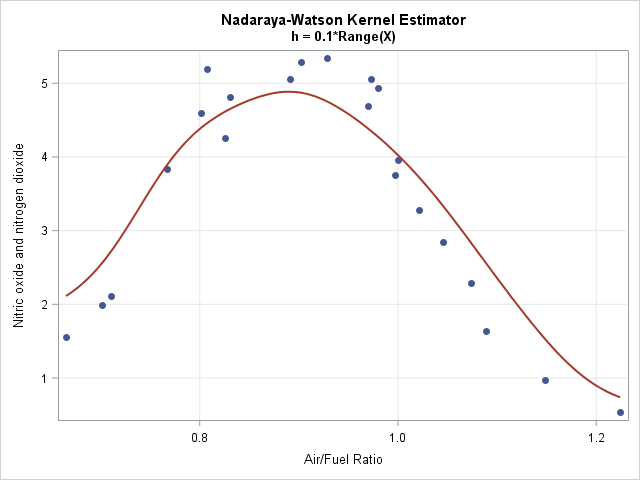If you use a degree-zero polynomial to compute the smoother, each predicted value is a locally-weighted average of the data. This smoother is called the Nadaraya–Watson (N-W) kernel estimator. Because the N-W smoother is a weighted average, the computation is much simpler than for linear regression. The following two modules show the main computation. The N-W kernel smoother on the same exhaust data is shown to the right.

```/* Nadaraya-Watson kernel estimator, which is a locally weighted average */ start NWKerReg(Y, X, x0, H); K = colvec( pdf("Normal", X, x0, H*range(X)) ); return( K`*Y / sum(K) ); finish;   /* Nadaraya-Watson kernel smoother module. (X,Y) are column vectors that contain the data. H is the proportion of the data range. It determines the bandwidth for the kernel regression as h = H*range(X) t is a vector of values at which to evaluate the fit. By default t=X. */ start NWKernelSmoother(X, Y, H, _t=X); t = colvec(_t); pred = j(nrow(t), 1, .); do i = 1 to nrow(t); pred[i] = NWKerReg(Y, X, t[i], H); end; return pred; finish;```

### Problems with kernel regression

As mentioned earlier, there are some intrinsic problems with kernel regression smoothers:

• Kernel smoothers treat extreme values of a sample distribution differently than points near the middle of the sample. For example, if x0 is the minimum value of the data, the fit at x0 is a weighted average of only the observations that are greater than x0. In contrast, if x0 is the median value of the data, the fit is a weighted average of points on both sides of x0. You can see the bias in the graph of the Nadaraya–Watson estimate, which shows predicted values that are higher than the actual values for the smallest and largest values of the X variable. Some researchers have proposed modified kernels to handle this problem for the N-W estimate. Fortunately, the local linear estimator tends to perform well.
• The bandwidth of kernel smoothers cannot be too small or else there are values of x0 for which prediction is not possible. If d = x[i+1] – x[i]is the gap between two consecutive X data values, then if h is smaller than about d/10, there is no way to predict a value at the midpoint (x[i+1] + x[i]) / 2. The linear system that you need to solve will be singular because the weights at x0 are zero. The loess method avoids this flaw by using nearest neighbors to form predicted values. A nearest-neighbor algorithm is a variable-width kernel method, as opposed to the fixed-width kernel used here.

### Extensions of the simple kernel smoother

You can extend the one-dimensional example to more complex situations:

• Higher Dimensions: You can compute kernel regression for two or more independent variables by using a multivariate normal density as the kernel function. In theory, you could use any correlated structure for the kernel function, but in practice most people use a covariance structure of the form Σ = diag(h21, ..., h2k), where hi is the bandwidth in the i_th coordinate direction. An even simpler covariance structure is to use the same bandwidth in all directions, which can be evaluated by using the one-dimensional standard normal density evaluated at || x - x0 || / h. Details are left as an exercise.
• Automatic selection of the bandwidth: The example in this article uses a specified bandwidth, but as I have written before, it is better to use a fit criterion such as GCV or AIC to select a smoothing parameter.

In summary, you can use a weighted polynomial regression to implement a kernel smoother in SAS. The weights are provided by a kernel density function, such as the normal density. Implementing a simple kernel smoother requires only a few statements in the SAS/IML matrix language. However, most modern data analysts prefer a loess smoother over a kernel smoother because the loess algorithm (which is a varying-width kernel algorithm) solves some of the issues that arise when trying to use a fixed-width kernel smoother with a small bandwidth. You can use PROC LOESS in SAS to compute a loess smoother.

The post Kernel regression in SAS appeared first on The DO Loop.A SAS programmer recently asked how to interpret the "standardized regression coefficients" as computed by the STB option on the MODEL statement in PROC REG and other SAS regression procedures. The SAS documentation for the STB option states, "a standardized regression coefficient is computed by dividing a parameter estimate by the ratio of the sample standard deviation of the dependent variable to the sample standard deviation of the regressor." Although correct, this definition does not provide an intuitive feeling for how to interpret the standardized regression estimates. This article uses SAS to demonstrate how parameter estimates for the original variables are related to parameter estimates for standardized variables. It also derives how regression coefficients change after a linear transformation of the variables.

### Proof by example

One of my college physics professors used to smile and say "I will now prove this by example" when he wanted to demonstrate a fact without proving it mathematically. This section uses PROC STDIZE and PROC REG to "prove by example" that the standardized regression estimates for data are equal to the estimates that you obtain by standardizing the data. The following example uses continuous response and explanatory variables, but there is a SAS Usage Note that describes how to standardize classification variables.

The following call to PROC REG uses the STB option to compute the standardized parameter estimates for a model that predicts the weights of 19 students from heights and ages:

```proc reg data=Sashelp.Class plots=none; Orig: model Weight = Height Age / stb; ods select ParameterEstimates; quit;```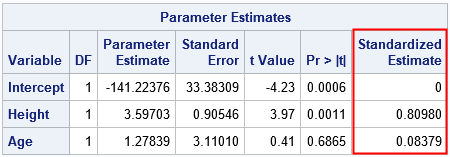The last column is the result of the STB option on the MODEL statement. You can get the same numbers by first standardizing the data and then performing a regression on the standardized variables, as follows:

```/* Put original and standardized variables into the output data set. Standardized variables have the names 'StdX' where X was the name of the original variable. METHOD=STD standardizes variables according to StdX = (X - mean(X)) / std(X) */ proc stdize data=Sashelp.Class out=ClassStd method=std OPREFIX SPREFIX=Std; run;   proc reg data=ClassStd plots=none; Std: model StdWeight = StdHeight StdAge; ods select ParameterEstimates; quit;```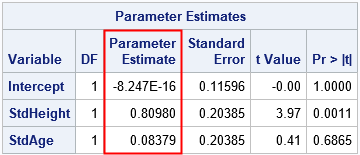The parameter estimates for the standardized data are equal to the STB estimates for the original data. Furthermore, the t values and p-values for the slope parameters are equivalent because these statistics are scale- and translation-invariant. Notice, however, that scale-dependent statistics such as standard errors and covariance of the betas will not be the same for the two analyses.

### Linear transformations of random variables

Mathematically, you can use a algebra to understand how linear transformations affect the relationship between two linearly dependent random variables. Suppose X is a random variable and Y = b0 + b1*X for some constants b0 and b1. What happens to this linear relationship if you apply linear transformations to X and Y?

Define new variables U = (Y - cY) / sY and V = (X - cX) / sX. If you solve for X and Y and plug the expressions into the equation for the linear relationship, you find that the new random variables are related by
U = (b0 + b1*cX - cY) / sY + b1*(sX/sY)*V.
If you define B0 = (b0 + b1*cX - cY) / sY and B1 = b1*(sX/sY), then U = B0 + B1*V, which shows that the transformed variables (U and V) are linearly related.

The physical significance of this result is that linear relationships persist no matter what units you choose to measure the variables. For example, if X is measured in inches and Y is measured in pounds, then the quantities remain linearly related if you measure X in centimeters and measure Y in "kilograms from 20 kg."

### The effect of standardizing variables on regression estimates

The analysis in the previous section holds for any linear transformation of linearly related random variables. But suppose, in addition, that

• U and V are standardized versions of Y and X, respectively. That is, cY and cX are the sample means and sY and sX are the sample standard deviations.
• The parameters b0 and b1 are the regression estimates for a simple linear regression model.

For simple linear regression, the intercept estimate is b0 = cY - b1*cY, which implies that B0 = 0. Furthermore, the coefficient B1 = b1*(sX/sY) is the original parameter estimate "divided by the ratio of the sample standard deviation of the dependent variable to the sample standard deviation of the regressor," just as the PROC REG documentation states and just as we saw in the PROC REG output in the previous section. Thus the STB option on the MODEL statement does not need to standardize any data! It produces the standardized estimates by setting the intercept term to 0 and dividing the parameter estimates by the ratio of standard deviations, as noted in the documentation. (A similar proof handles multiple explanatory variables.)

### Interpretation of the regression coefficients

For the original (unstandardized) data, the intercept estimate predicts the value of the response when the explanatory variables are all zero. The regression coefficients predict the change in the response for one unit change in an explanatory variable. The "change in response" depends on the units for the data, such as kilograms per centimeter.

The standardized coefficients predict the number of standard deviations that the response will change for one STANDARD DEVIATION of change in an explanatory variable. The "change in response" is a unitless quantity. The fact that the standardized intercept is 0 indicates that the predicted value of the (centered) response is 0 when the model is evaluated at the mean values of the explanatory variables.

In summary, standardized coefficients are the parameter estimates that you would obtain if you standardize the response and explanatory variables by centering and scaling the data. A standardized parameter estimate predicts the change in the response variable (in standard deviations) for one standard deviation of change in the explanatory variable.

The post Standardized regression coefficients appeared first on The DO Loop.Video killed the radio star....
We can't rewind, we've gone too far.
-- The Buggles (1979)

"You kids have it easy," my father used to tell me. "When I was a kid, I didn't have all the conveniences you have today." He's right, and I could say the same thing to my kids, especially about today's handheld technology. In particular, I've noticed that powerful handheld technology (especially the modern calculator) has killed the standard probability tables that were once ubiquitous in introductory statistics textbooks.

In my first probability and statistics course, I constantly referenced the 23 statistical tables (which occupied 44 pages!) in the appendix of my undergraduate textbook. Any time I needed to compute a probability or test a hypothesis, I would flip to a table of probabilities for the normal, t, chi-square, or F distribution and use it to compute a probability (area) or quantile (critical value). If the value I needed wasn't tabulated, I had to manually perform linear interpolation from two tabulated values. I had no choice: my calculator did not have support for these advanced functions.

In contrast, kids today have it easy! When my son took AP statistics in high school, his handheld calculator (a TI-84, which costs about \$100) could compute the PDF, CDF, and quantiles of all the important probability distributions. Consequently, his textbook did not include an appendix of statistical tables.

It makes sense that publishers would choose to omit these tables, just as my own high school textbooks excluded the trig and logarithm tables that were prevalent in my father's youth. When handheld technology can reproduce all the numbers in a table, why waste the ink and paper?

In fact, by using SAS software, you can generate and display a statistical table with only a few statements. To illustrate this, let's use SAS to generate two common statistical tables: a normal probability table and a table of critical values for the chi-square statistic.

### A normal probability table

A normal probability table gives an area under the standard normal density curve. As discussed in a Wikipedia article about the standard normal table, there are three equivalent kinds of tables, but I will use SAS to produce the first table on the list. Given a standardized z-score, z > 0, the table gives the probability that a standard normal random variate is in the interval (0, z). That is, the table gives P(0 < Z < z) for a standard normal random variable Z. The graph below shows the shaded area that is given in the body of the table.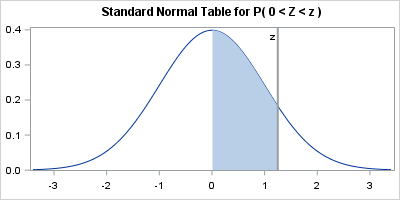You can create the table by using the SAS DATA step, but I'll use SAS/IML software. The rows of the table indicate the z-score to the first decimal place. The columns of the table indicate the second decimal place of the z-score. The key to creating the table is to recall that you can call any Base SAS function from SAS/IML, and you can use vectors of parameters. In particular, the following statements use the EXPANDGRID function to generate all two-decimal z-scores in the range [0, 3.4]. The program then calls the CDF function to evaluate the probability P(Z < z) and subtracts 1/2 to obtain the probability P(0 < Z < z). The SHAPE function reshapes the vector of probabilities into a 10-column table. Finally, the PUTN function converts the column and row headers into character values that are printed at the top and side of the table.

```proc iml; /* normal probability table similar to https://en.wikipedia.org/wiki/Standard_normal_table#Table_examples */ z1 = do(0, 3.4, 0.1); /* z-score to first decimal place */ z2 = do(0, 0.09, 0.01); /* second decimal place */ z = expandgrid(z1, z2)[,+]; /* sum of all pairs from of z1 and z2 values */ p = cdf("Normal", z) - 0.5; /* P( 0 < Z < z ) */ Prob = shape( p, ncol(z1) ); /* reshape into table with 10 columns */   z1Lab = putn(z1, "3.1"); /* formatted values of z1 for row headers */ z2Lab = putn(z2, "4.2"); /* formatted values of z2 for col headers */ print Prob[r=z1Lab c=z2Lab F=6.4 L="Standard Normal Table for P( 0 < Z < z )"];```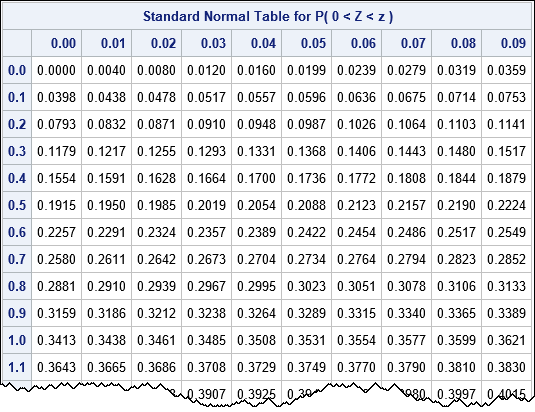To find the probability between 0 and z=0.67, find the row for z=0.6 and then move over to the column labeled 0.07. The value of that cell is P(0 < Z < 0.67) = 0.2486.

### Chi-square table of critical values

Some statistical tables display critical values for a test statistic instead of probabilities. This section shows how to construct a table of the critical values of a chi-square test statistic for common significance levels (α). The rows of the table correspond to degrees of freedom; the columns correspond to significance levels. The following graph shows the situation. The shaded area corresponds to significance levels.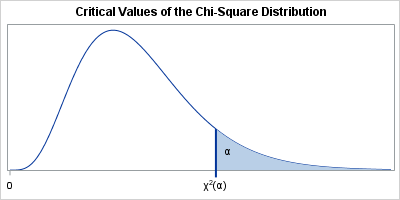The corresponding table provides the quantile of the distribution that corresponds to each significance level. Again, you can use the DATA step, but I have chosen to use SAS/IML software to generate the table:

```/* table of critical values for the chi-square distribution https://flylib.com/books/3/287/1/html/2/images/xatab02.jpg */ df = (1:30) || do(40,100,10); /* degrees of freedom */ /* significance levels for common one- or two-sided tests */ alpha = {0.99 0.975 0.95 0.9 0.1 0.05 0.025 0.01}; g = expandgrid(df, alpha); /* all pairs of (df, alpha) values */ p = quantile("ChiSquare", 1 - g[,2], g[,1]); /* compute quantiles for (df, 1-alpha) */ CriticalValues = shape( p, ncol(df) ); /* reshape into table */   dfLab = putn(df, "3."); /* formatted row headers */ pLab = putn(alpha, "5.3"); /* formatted col headers */ print CriticalValues[rowname=dfLab colname=pLab F=6.3 L="Critical Values of Chi-Square Distribution"];```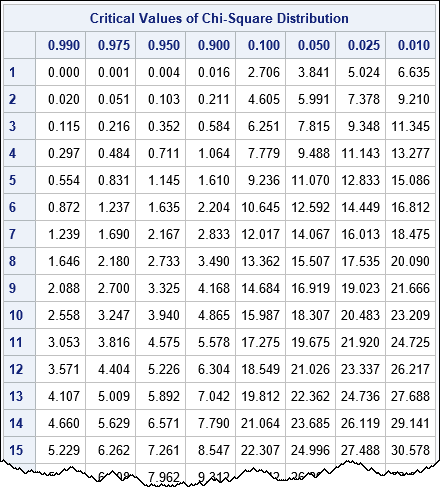To illustrate how to use the table, suppose that you have computed a chi-square test statistic for 9 degrees of freedom. You want to determine if you should reject the (one-sided) null hypothesis at the α = 0.05 significance level. You trace down the table to the row for df=9 and then trace over to the column for 0.05. The value in that cell is 16.919, so you would reject the null hypothesis if your test statistic exceeds that critical value.

Just as digital downloads of songs have supplanted records and CDs, so, too, have modern handheld calculators replaced the statistical tables that used to feature prominently in introductory statistics courses. However, if you ever feel nostalgic for the days of yore, you can easily resurrect your favorite table by writing a few lines of SAS code. To be sure, there are some advanced tables (the Kolmogorov-Smirnov test comes to mind) that have not yet been replaced by a calculator key, but the simple tables are dead. Killed by the calculator.

It might be ill to speak of the dead, but I say, "good riddance"; I never liked using tables those tables anyway. What are your thoughts? If you are old enough to remember tables do you have fond memories of using them? If you learned statistics recently, are you surprised that tables were once so prevalent? Share your experiences by leaving a comment.

The post Calculators killed the standard statistical table appeared first on The DO Loop.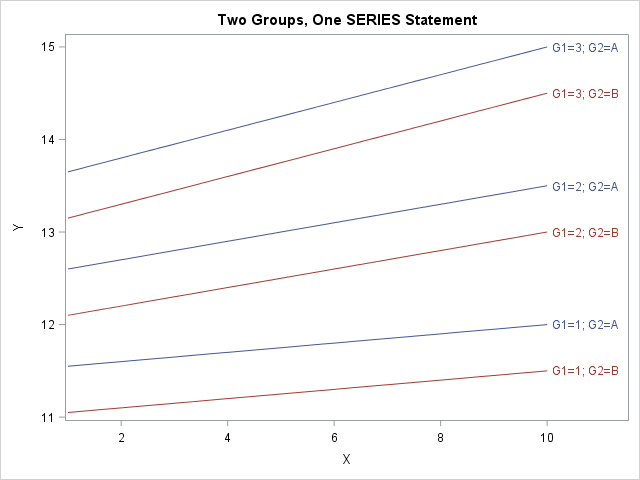The SGPLOT procedure in SAS makes it easy to create graphs that overlay various groups in the data. Many statements support the GROUP= option, which specifies that the graph should overlay group information. For example, you can create side-by-side bar charts and box plots, and you can overlay multiple scatter plots and series plots in the same graph. However, the GROUP= option takes only a single grouping variable! What can you do if you need to visualize combinations of two (or even three!) categorical variables? This article shows how to construct a new group variable that combines the levels of two or more existing categorical variables.

For concreteness, I will show how to overlay multiple series plots (line plots), as shown to the right. (Click to enlarge.) By using this technique, you can overlay curves that describe combinations of gender and race. Or you can plot response curves for control-vs-experimental groups and the severity of a disease. You can use this technique for other plot types, such as creating box plots that visualize a two-way ANOVA.

### What is the problem? Why can't you use the GROUP= option?

To motivate the discussion, let's first see why the GROUP= option in the SERIES statement does not work for overlaying two categorical variables. The following DATA step creates two categorical variables. The G1 variable has the values 1, 2, and 3. The G2 variable has the values 'A' and 'B'. For each of the six combinations of (G1, G2), the DATA step creates a curve (actually a line) of (X, Y) values. Let's see what happens if you try to plot the lines by using a SERIES statement with the GROUP=G1 option:

```data TwoGroups; do G1=1 to 3; do G2='A', 'B'; do X = 1 to 10; Y = 10 + G1 + 0.5*(G2='A') + G1*X/20; output; end; end; end; run;   title "First Attempt: Does Not Work"; proc sgplot data=TwoGroups; series x=x y=y / group=G1 curvelabel; run;```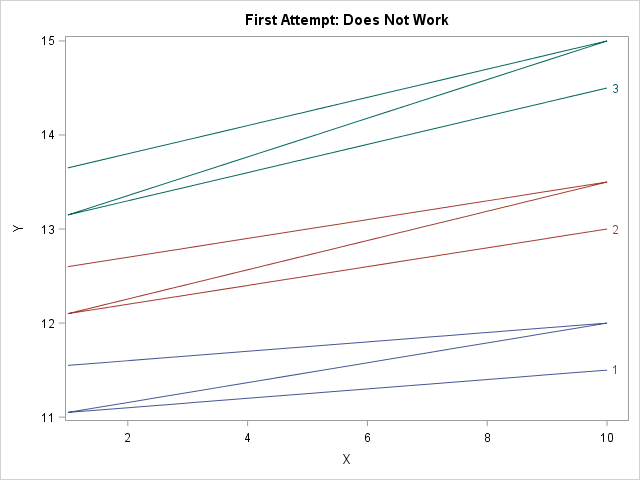The attempt is a failure. The graph does not show six individual curves. Instead, it shows three curves (the number of categories in the G1 variables) and each "curve" is Z-shaped because the graph traces the curve for G2='A' on the range [1, 10] and then draws the curve for G2='B' without "picking up the pen." This happens because the data are sorted by G1, then by G2, then by X.

There are two ways to handle this situation. One way is to try to convert the data from "long form" to "wide form." For these data, which have identical X values for every curve, you can create two new variables Y_A and Y_B that contain the coordinates of the three curves for G2='A' and G2='B', respectively. You can then use two SERIES statements, each using the GROUP=G1 option. Each statement will draw three curves for a total of six. You can use the NOCYCLEATTRS option to make sure that each statement uses the same line colors and patterns. However, this approach becomes complicated if each curve is evaluated at a different set of X values. In that case, it is better to keep the data in long form.

### Forming a new group variable by concatenation

The problem would be solved if there were one categorical variable that had six levels instead of two categorical variables that have six joint levels, so let's write some SAS code to make that happen. First sort the data by the categorical variables and then by the X variable. (For the current example, the data are already sorted correctly.) Then write a DATA step that does either of the following options:

• Option 1: Use the CATT (or CATX) function to concatenate the values of the existing group variables. The new categorical variable will have values that derived from the original variables.
• Option 2: Use the FIRST.variable syntax to create a new group variable that has the values 1–6. Then use PROC FORMAT to assign a meaningful value to each level of the new categorical variable.

The first option (the CATT function) is automated and less prone to error, but for the sake of completeness both options are shown below:

```/* create a new group variable by concatenating the two existing variables */ proc sort data=TwoGroups; by G1 G2 X; run;   data Make2Groups; set TwoGroups; by G1 G2; /* Option 1: automatically create joint levels from original levels */ Label = catt("G1=", G1) || "; " || catt("G2=", G2); /* Option 2: create new categorical variable and use PROC FORMAT to assign values */ if first.G2 then GroupID + 1; /* GroupID = 1, 2, 3, ... numGroups */ run;   /* For Option 2: use PROC FORMAT to form labels that encode the two groups. See https://blogs.sas.com/content/iml/2017/04/24/two-way-anova-viz.html */ proc format; value GroupFmt 1 = "G1=1; G2='A'" 2 = "G1=1; G2='B'" 3 = "G1=2; G2='A'" 4 = "G1=2; G2='B'" 5 = "G1=3; G2='A'" 6 = "G1=3; G2='B'"; run;```

The following call to PROC SGPLOT creates a series plot for the Label variable, which corresponds to the joint levels of the original grouping variables. The GROUPLC= option (supported in SAS 9.4M2) colors the lines according to the value of the G2 variable.

```title "Two Groups, One SERIES Statement"; proc sgplot data=Make2Groups; /* format GroupID GroupFmt.; */ /* for Option 2 */ series x=x y=y / group=Label grouplc=G2 /* or group=GroupID for Option 2 */ lineattrs=(pattern=solid) curvelabel; run;```

The graph is shown at the top of this article. The new categorical variable has values that correspond to joint levels of the original two variables. The GROUP= option creates six curves when you specify the Label variable. The GROUPLC= option sets the colors of the lines according to the values of the G2 variable.

This technique generalizes to three categorical variables, but I will leave the details to the reader. You might want to use the GROUPLP= option to set the line patterns according to the value of a third categorical variable. Beyond three variables the display will begin to resemble a spaghetti plot. For many categorical variables, you might want to use panels and BY groups to visualize the curves.

This technique also generalizes to other plot types, such as box plots and scatter plots.

The post Plot curves for levels of two categorical variables in SAS appeared first on The DO Loop.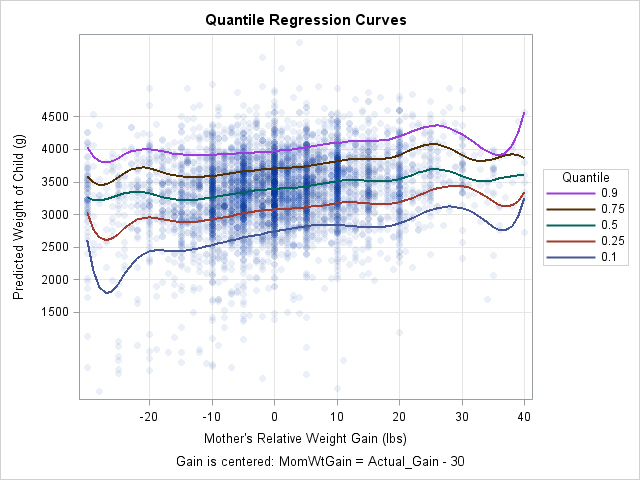This article shows how to score (evaluate) a quantile regression model on new data. SAS supports several procedures for quantile regression, including the QUANTREG, QUANTSELECT, and HPQUANTSELECT procedures. The first two procedures do not support any of the modern methods for scoring regression models, so you must use the "missing value trick" to score the model. (HPQUANTSELECT supports the CODE statement for scoring.) You can use this technique to construct a "sliced fit plot" that visualizes the model, as shown to the right. (Click to enlarge.)

### The easy way to create a fit plot

The following DATA step creates the example data as a subset of the Sashelp.BWeight data set, which contains information about the weights of live births in the US in 1997 and information about the mother during pregnancy. The following call to PROC QUANTREG models the conditional quantiles of the baby's weight as a function of the mother's weight gain. The weight gain is centered according to the formula MomWtGain = "Actual Gain" – 30 pounds. Because the quantiles might depend nonlinearly on the mother's weight gain, the EFFECT statement generates a spline basis for the independent variable. The resulting model can flexibly fit a wide range of shapes.

Although this article shows how to create a fit plot, you can also get a fit plot directly from PROC QUANTREG. As shown below, the PLOT=FITPLOT option creates a fit plot when the model contains one continuous independent variable.

```data Orig; /* restrict to 5000 births; exclude extreme weight gains */ set Sashelp.BWeight(obs=5000 where=(MomWtGain<=40)); run;   proc quantreg data=Orig algorithm=IPM /* use IPM algorithm for splines and binned data */ ci=none plot(maxpoints=none)=fitplot; /* or fitplot(nodata) */ effect MomWtSpline = spline( MomWtGain / knotmethod = equal(9) ); /* 9 knots, equally spaced */ model Weight = MomWtSpline / quantile = 0.1 0.25 0.5 0.75 0.90; run;```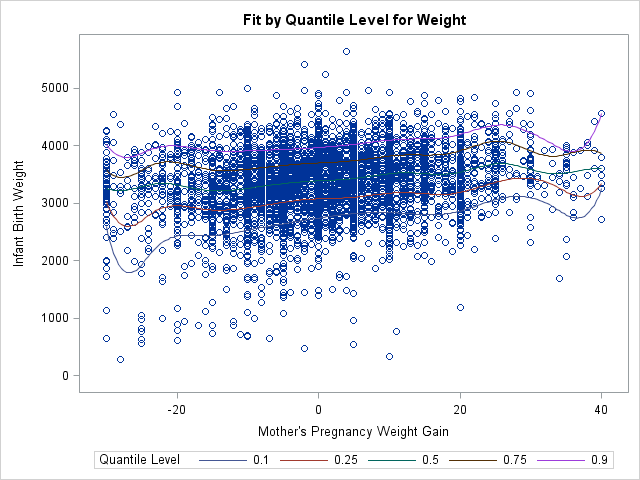The graph enables you to visualize curves that predict the 10th, 25th, 50th, 75th, and 90th percentiles of the baby's weight based on the mother's weight gain during pregnancy. Because the data contains 5,000 observations, the fit plot suffers from overplotting and the curves are hard to see. You can use the PLOT=FITPLOT(NODATA) option to exclude the data from the plot, thus showing the quantile curves more clearly.

### Score a SAS procedure by using the missing value trick

Although PROC QUANTREG can produce a fit plot when there is one continuous regressor, it does not support the EFFECTPLOT statement so you have to create more complicated graphs manually. To create a graph that shows the predicted values, you need to score the model on a new set of independent values. To use the missing value trick, do the following:

1. Create a SAS data set (the scoring data) that contains the values of the independent variables at which you want to evaluate the model. Set the response variable to missing for each observation.
2. Append the scoring data to the original data that are used to fit the model. Include a binary indicator variable that has the value 0 for the original data and the value 1 for the scoring data.
3. Run the regression procedure on the combined data set. Use the OUTPUT statement to output the predicted values for the scoring data. Of course, you can also output residuals and other observation-wise statistics, if necessary.

This general technique is implemented by using the following SAS statements. The scoring data consists of evenly spaced values of the MomWtGain variable. The binary indicator variable is named ScoreData. The result of these computations is a data set named QRegOut that contains a variable named Pred that contains the predicted values for each observation in the scoring data.

```/* 1. Create score data set */ data Score; /* Optionally define additional covariates here. See the example "Create a sliced fit plot manually by using the missing value trick" https://blogs.sas.com/content/iml/2017/12/20/create-sliced-fit-plot-sas.html */ Weight = .; /* set response (Y) variable to missing */ do MomWtGain = -30 to 40; /* uniform spacing in the independent (X) variable */ output; end; run;   /* 2. Append the score data to the original data. Use a binary variable to indicate which observations are the scoring data */ data Combined; set Orig /* original data */ Score(in=_ScoreData); /* scoring data */ ScoreData = _ScoreData; /* binary indicator variable. ScoreData=1 for scoring data */ run;   /* 3. Run the procedure on the combined (original + scoring) data */ ods select ModelInfo NObs; proc quantreg data=Combined algorithm=IPM ci=none; effect MomWtSpline = spline( MomWtGain / knotmethod = equal(9) ); model Weight = MomWtSpline / quantile = 0.1 0.25 0.5 0.75 0.90; output out=QRegOut(where=(ScoreData=1)) /* output predicted values for the scoring data */ P=Pred / columnwise; /* COLUMWISE option supports multiple quantiles */ run;```

This technique can be used for any SAS regression procedure. In this case, the COLUMNWISE option specifies that the output data set should be written in "long form": A QUANTILE variable specifies the quantile and the variable PRED contains the predicted values for each quantile. If you omit the COLUMNWISE option, the output data is in "wide form": The predicted values for the five quantiles are contained in the variables Pred1, Pred2, ..., Pred5.

### Using predicted values to create a sliced fit plot

You can use the predicted values of the scoring data to construct a fit plot. You merely need to sort the data by any categorical variables and by the X variable (in this case, MomWtGain). You can then plot the predicted curves. If desired, you can also append the original data and the predicted values and create a graph that overlays the data and predicted curves. You can use transparency to address the overplotting issue and also modify other features of the fit plot, such as the title, axes labels, tick positions, and so forth:

```/* 4. If you want a fit plot, sort by the independent variable for each curve. Put QUANTILE and other covariates first, then the X variable. */ proc sort data=QRegOut out=ScoreData; by Quantile MomWtGain; run;   /* 5. (optional) If you want to overlay the plots, it's easiest to define separate variables for original data and scoring data */ data All; set Orig /* original data */ ScoreData(rename=(MomWtGain=X Pred=Y)); /* scoring data */ run;   title "Quantile Regression Curves"; footnote J=C "Gain is centered: MomWtGain = Actual_Gain - 30"; proc sgplot data=All; scatter x=MomWtGain y=Weight / markerattrs=(symbol=CircleFilled) transparency=0.92; series x=X y=Y / group=Quantile lineattrs=(thickness=2) nomissinggroup name="p"; keylegend "p" / position=right sortorder=reverseauto title="Quantile"; xaxis values=(-20 to 40 by 10) valueshint grid label="Mother's Relative Weight Gain (lbs)";; yaxis values=(1500 to 4500 by 500) valueshint grid label="Predicted Weight of Child (g)"; run;```

The fit plot is shown at the top of this article.

In summary, this article shows how to use the missing value trick to evaluate a regression model in SAS. You can use this technique for any regression procedure, although newer procedures often support syntax that makes it easier to score a model.

As shown in this example, if you score the model on an equally spaced set of points for one of the continuous variables in the model, you can create a sliced fit plot. For a more complicated example, see the article "How to create a sliced fit plot in SAS."

The post How to score and graph a quantile regression model in SAS appeared first on The DO Loop.This article shows how to score (evaluate) a quantile regression model on new data. SAS supports several procedures for quantile regression, including the QUANTREG, QUANTSELECT, and HPQUANTSELECT procedures. The first two procedures do not support any of the modern methods for scoring regression models, so you must use the "missing value trick" to score the model. (HPQUANTSELECT supports the CODE statement for scoring.) You can use this technique to construct a "sliced fit plot" that visualizes the model, as shown to the right. (Click to enlarge.)

### The easy way to create a fit plot

The following DATA step creates the example data as a subset of the Sashelp.BWeight data set, which contains information about the weights of live births in the US in 1997 and information about the mother during pregnancy. The following call to PROC QUANTREG models the conditional quantiles of the baby's weight as a function of the mother's weight gain. The weight gain is centered according to the formula MomWtGain = "Actual Gain" – 30 pounds. Because the quantiles might depend nonlinearly on the mother's weight gain, the EFFECT statement generates a spline basis for the independent variable. The resulting model can flexibly fit a wide range of shapes.

Although this article shows how to create a fit plot, you can also get a fit plot directly from PROC QUANTREG. As shown below, the PLOT=FITPLOT option creates a fit plot when the model contains one continuous independent variable.

```data Orig; /* restrict to 5000 births; exclude extreme weight gains */ set Sashelp.BWeight(obs=5000 where=(MomWtGain<=40)); run;   proc quantreg data=Orig algorithm=IPM /* use IPM algorithm for splines and binned data */ ci=none plot(maxpoints=none)=fitplot; /* or fitplot(nodata) */ effect MomWtSpline = spline( MomWtGain / knotmethod = equal(9) ); /* 9 knots, equally spaced */ model Weight = MomWtSpline / quantile = 0.1 0.25 0.5 0.75 0.90; run;```The graph enables you to visualize curves that predict the 10th, 25th, 50th, 75th, and 90th percentiles of the baby's weight based on the mother's weight gain during pregnancy. Because the data contains 5,000 observations, the fit plot suffers from overplotting and the curves are hard to see. You can use the PLOT=FITPLOT(NODATA) option to exclude the data from the plot, thus showing the quantile curves more clearly.

### Score a SAS procedure by using the missing value trick

Although PROC QUANTREG can produce a fit plot when there is one continuous regressor, it does not support the EFFECTPLOT statement so you have to create more complicated graphs manually. To create a graph that shows the predicted values, you need to score the model on a new set of independent values. To use the missing value trick, do the following:

1. Create a SAS data set (the scoring data) that contains the values of the independent variables at which you want to evaluate the model. Set the response variable to missing for each observation.
2. Append the scoring data to the original data that are used to fit the model. Include a binary indicator variable that has the value 0 for the original data and the value 1 for the scoring data.
3. Run the regression procedure on the combined data set. Use the OUTPUT statement to output the predicted values for the scoring data. Of course, you can also output residuals and other observation-wise statistics, if necessary.

This general technique is implemented by using the following SAS statements. The scoring data consists of evenly spaced values of the MomWtGain variable. The binary indicator variable is named ScoreData. The result of these computations is a data set named QRegOut that contains a variable named Pred that contains the predicted values for each observation in the scoring data.

```/* 1. Create score data set */ data Score; /* Optionally define additional covariates here. See the example "Create a sliced fit plot manually by using the missing value trick" https://blogs.sas.com/content/iml/2017/12/20/create-sliced-fit-plot-sas.html */ Weight = .; /* set response (Y) variable to missing */ do MomWtGain = -30 to 40; /* uniform spacing in the independent (X) variable */ output; end; run;   /* 2. Append the score data to the original data. Use a binary variable to indicate which observations are the scoring data */ data Combined; set Orig /* original data */ Score(in=_ScoreData); /* scoring data */ ScoreData = _ScoreData; /* binary indicator variable. ScoreData=1 for scoring data */ run;   /* 3. Run the procedure on the combined (original + scoring) data */ ods select ModelInfo NObs; proc quantreg data=Combined algorithm=IPM ci=none; effect MomWtSpline = spline( MomWtGain / knotmethod = equal(9) ); model Weight = MomWtSpline / quantile = 0.1 0.25 0.5 0.75 0.90; output out=QRegOut(where=(ScoreData=1)) /* output predicted values for the scoring data */ P=Pred / columnwise; /* COLUMWISE option supports multiple quantiles */ run;```

This technique can be used for any SAS regression procedure. In this case, the COLUMNWISE option specifies that the output data set should be written in "long form": A QUANTILE variable specifies the quantile and the variable PRED contains the predicted values for each quantile. If you omit the COLUMNWISE option, the output data is in "wide form": The predicted values for the five quantiles are contained in the variables Pred1, Pred2, ..., Pred5.

### Using predicted values to create a sliced fit plot

You can use the predicted values of the scoring data to construct a fit plot. You merely need to sort the data by any categorical variables and by the X variable (in this case, MomWtGain). You can then plot the predicted curves. If desired, you can also append the original data and the predicted values and create a graph that overlays the data and predicted curves. You can use transparency to address the overplotting issue and also modify other features of the fit plot, such as the title, axes labels, tick positions, and so forth:

```/* 4. If you want a fit plot, sort by the independent variable for each curve. Put QUANTILE and other covariates first, then the X variable. */ proc sort data=QRegOut out=ScoreData; by Quantile MomWtGain; run;   /* 5. (optional) If you want to overlay the plots, it's easiest to define separate variables for original data and scoring data */ data All; set Orig /* original data */ ScoreData(rename=(MomWtGain=X Pred=Y)); /* scoring data */ run;   title "Quantile Regression Curves"; footnote J=C "Gain is centered: MomWtGain = Actual_Gain - 30"; proc sgplot data=All; scatter x=MomWtGain y=Weight / markerattrs=(symbol=CircleFilled) transparency=0.92; series x=X y=Y / group=Quantile lineattrs=(thickness=2) nomissinggroup name="p"; keylegend "p" / position=right sortorder=reverseauto title="Quantile"; xaxis values=(-20 to 40 by 10) valueshint grid label="Mother's Relative Weight Gain (lbs)";; yaxis values=(1500 to 4500 by 500) valueshint grid label="Predicted Weight of Child (g)"; run;```

The fit plot is shown at the top of this article.

In summary, this article shows how to use the missing value trick to evaluate a regression model in SAS. You can use this technique for any regression procedure, although newer procedures often support syntax that makes it easier to score a model.

As shown in this example, if you score the model on an equally spaced set of points for one of the continuous variables in the model, you can create a sliced fit plot. For a more complicated example, see the article "How to create a sliced fit plot in SAS."

The post How to score and graph a quantile regression model in SAS appeared first on The DO Loop.